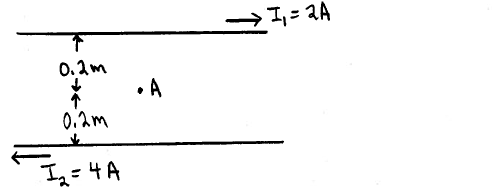🤓 Based on our data, we think this question is relevant for Professor Laird's class at FIU.

# Solution: Two long straight parallel wires carry currents as shown in the sketch. Point  A is midway between the two wires, 0.20 m from each wire. What are the magnitude and direction of the net magnetic field at point A due to the two wires?

###### Problem

Two long straight parallel wires carry currents as shown in the sketch. Point  A is midway between the two wires, 0.20 m from each wire. What are the magnitude and direction of the net magnetic field at point A due to the two wires?